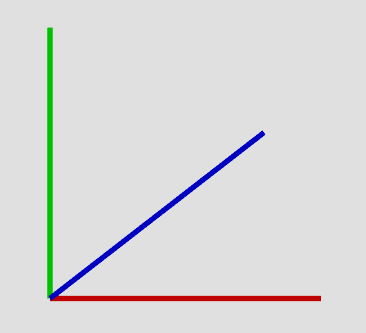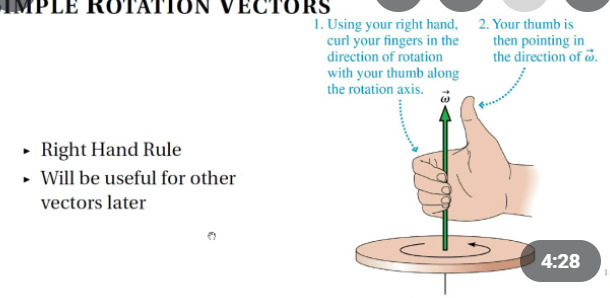# Keep orientation with BodyAngularVelocity

Firstly, i won’t use `BodyGyro` because it’s too rigid im my opinion (along with other bugs that occured). This will be used in a “custom character movement” type thing, and i want it to be HEAVILY physics based.
On to the question at hand:

How can i keep an orientation with `BodyAngularVelocity`?
I think the best way of doing this is by aligning vectors (Up, Look, Left vectors). AKA: Calculate the difference and somehow convert it to angular velocity.
But as to how? I have almost no clue.

Example problem:
I have a green and a red block. I need to align red’s orientation to greens’s.

1. I have to calculate the difference in rotation.
2. I have to use this difference to calculate angular velocity.

How would i go about writing this in code?
Any help is appreciated.

You can calculate the difference in vectors using the advanced CFrame trick:

particularly:

``````local function getRotationBetween(u, v, axis)
local dot, uxv = u:Dot(v), u:Cross(v)
if (dot < -0.99999) then return CFrame.fromAxisAngle(axis, math.pi) end
return CFrame.new(0, 0, 0, uxv.x, uxv.y, uxv.z, 1 + dot)
end
``````

So you can do stuff like align axises you can imagine the red vector is the unaligned humanoid resting on the floor and the green vector is the world Up Vector.However the newer Align orientation already supports for that with the primary axis only option:

This will make it “Less rigid” than BodyGyro as it is able to rotate freely in an axis and be less work.

Also I did something similar, of converting a CFrame to an angular velocity though it’s all CFrames.

1 Like

use AlignOrientation i think it works and much easier than calculating all the math yourself

@dthecoolest @Creeperman16487
AlignOrientation needs 2 attachment points, and i need this to be self contained with no workarounds. Let me tell you how i plan to implement:

There is a block and an imaginary CFrame. The white block is the character, the red block is the target CFrame. The red block is imaginary.

I don’t want a workaround like: Create a block with the CFrame, put attachments in it and use AlignOrientation.

I hope you see why i wanted it the way i said.

Not really, I don’t see the big issue as you can just parent the attachment to terrain and it will not disrupt anything else in the workspace.

But if you still want to know how to calculate it you use this function as I have said previously.

``````local function getRotationBetween(u, v, axis)
local dot, uxv = u:Dot(v), u:Cross(v)
if (dot < -0.99999) then return CFrame.fromAxisAngle(axis, math.pi) end
return CFrame.new(0, 0, 0, uxv.x, uxv.y, uxv.z, 1 + dot)
end
``````

You convert this cframe to axis angle representation which can represent angular velocity as well:

``````local axis, angle= getRotationBetween(humanoidRootPart.CFrame.UpVector, Vector3.new(0,1,0), axis):ToAxisAngle()
local angularDifferenceDistance = axis*angle --how much radians it will need to rotate on this axis before the vectors are aligned
``````

The issue is you will also need to use PID in order to stabilize the amount of force in order to not overshoot the goal too much and oscillate like crazy.

This is what align orientation solves for you already.

Another method to calculate the full difference in orientation is to use to object space

``````local partCF = part.CFrame
local targetCF = target.CFrame
local differenceCF = partCF:ToObjectSpace(targetCF)
local axis, angle = differenceCF:ToAxisAngle()
--obtain rotation difference in form of torque
``````
1 Like

I see what you mean. Let me process this first, my head is still too dense to this, even though i’ve been doing this for 6 months.Thank you tho.

So if i understand correctly… `angle` is the total difference in radians, and the `axis` is on what axis it differs.

Am i correct?

It represents the displacement rotational vector from Part1 to part2. Axis is where the thumb is pointing, angle is the amount of rotation that needs to be applied in the curling fingers direction to reach the goal orientation.But there are 3 axes. So each axis in the `axis` `Vector3` is essentially a “thumb”, right? (going by the image). And the values in them are the rotations that need to be applied.

You can decompose the angular differences between two CFrames using Euler methods. In this example I make two parts, one slightly rotated, then use the Euler method to reverse calculate the angles between the two.

``````local part1 = Instance.new("Part")
part1.Position = Vector3.new(0, 5, -20)
part1.Anchored = true
part1.Parent = game.Workspace

local part2 = Instance.new("Part")
part2.Position = Vector3.new(0, 5, -10)
I ended up multiplying the `axis` and `angle` together and setting it as the `AngularVelocity`. Works flawlessyl. And now i know more about `CFrames` (and this type of math).